• ## 对数函数

万次阅读 2019-11-10 22:27:37
一般地，对数函数是以幂（真数）为自变量，指数为因变量，底数为常量的函数。 对数函数是6类基本初等函数之一。其中对数的定义： 如果ax=N（a>0，且a≠1），那么数x叫做以a为底N的对数，记作x=logaN，读作以a为...
简介

一般地，对数函数是以幂（真数）为自变量，指数为因变量，底数为常量的函数。

对数函数是6类基本初等函数之一。其中对数的定义：

如果ax =N（a>0，且a≠1），那么数x叫做以a为底N的对数，记作x=logaN，读作以a为底N的对数，其中a叫做对数的底数，N叫做真数。

一般地，函数y=logaX（a>0，且a≠1）叫做对数函数，也就是说以幂（真数）为自变量，指数为因变量，底数为常量的函数，叫对数函数。

其中x是自变量，函数的定义域是（0，+∞），即x>0。它实际上就是指数函数的反函数，可表示为x=ay。因此指数函数里对于a的规定，同样适用于对数函数。

在实数域中，真数式子没根号那就只要求真数式大于零，如果有根号，要求真数大于零还要保证根号里的式子大于等于零（若为负数，则值为虚数），底数则要大于0且不为1。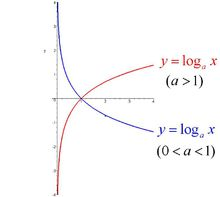对数函数

对数函数的底数为什么要大于0且不为1？【在一个普通对数式里 a<0，或=1 的时候是会有相应b的值。但是，根据对数定义：log以a为底a的对数；如果a=1或=0那么log以a为底a的对数就可以等于一切实数（比如log11也可以等于2，3，4，5，等等）】

通常我们将以10为底的对数叫常用对数（common logarithm），并把log10N记为lgN。另外，在科学计数中常使用以无理数e=2.71828···为底数的对数，以e为底的对数称为自然对数（natural logarithm），并且把logeN 记为In N。根据对数的定义，可以得到对数与指数间的关系：

当a>0，a≠1时，aX=N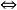X=logaN。（N>0）

由指数函数与对数函数的这个关系，可以得到关于对数的如下结论：

在实数范围内，负数和零没有对数；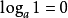，log以a为底1的对数为0（a为常数） 恒过点（1，0）。

有理和无理指数

如果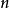是正整数，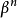表示等于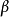的个因子的加减: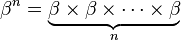加减

但是，如果是不等于1的正实数，这个定义可以扩展到在一个域中的任何实数（参见幂）。类似的，对数函数可以定义于任何正实数。对于不等于1的每个正底数，有一个对数函数和一个指数函数，它们互为反函数。

对数可以简化乘法运算为加法，除法为减法，幂运算为乘法，根运算为除法。所以，在发明电子计算机之前，对数对进行冗长的数值运算是很有用的，它们广泛的用于天文、工程、航海和测绘等领域中。它们有重要的数学性质而在今天仍在广泛使用中。

复对数

复对数计算公式

复数的自然对数，实部等于复数的模的自然对数，虚部等于复数的辐角。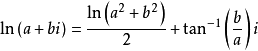产生历史

编辑

16世纪末至17世纪初的时候，当时在自然科学领域（特别是天文学）的发展上经常遇到大量精密而又庞大的数值计算，于是数学家们为了寻求化简的计算方法而发明了对数   。

德国的史蒂非（1487-1567）在1544年所著的《整数算术》中，写出了两个数列，左边是等比数列（叫原数），右边是一个等差数列（叫原数的代表，或称指数，德文是Exponent ，有代表之意）。

欲求左边任两数的积（商），只要先求出其代表（指数）的和（差），然后再把这个和（差）对向左边的一个原数，则此原数即为所求之积（商），可惜史提非并未作进一步探索，没有引入对数的概念。

纳皮尔对数值计算颇有研究。他所制造的「纳皮尔算筹」，化简了乘除法运算，其原理就是用加减来代替乘除法。 他发明对数的动机是为寻求球面三角计算的简便方法，他依据一种非常独等的与质点运动有关的设想构造出所谓对数方法，其核心思想表现为算术数列与几何数列之间的联系。在他的1619年发表《奇妙的对数表的描述》中阐明了对数原理，后人称为 纳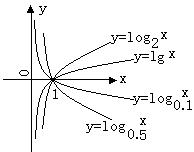对数的图像

皮尔对数，记为Nap．㏒x，它与自然对数的关系为：

Nap．㏒x=10㏑（107/x）

由此可知，纳皮尔对数既不是自然对数，也不是常用对数，与现今的对数有一定的距离。

瑞士的彪奇（1552-1632）也独立地发现了对数，可能比纳皮尔较早，但发表较迟（1620）。

英国的布里格斯在1624年创造了常用对数。

1619年，伦敦斯彼得所著的《新对数》使对数与自然对数更接近（以e=2.71828...为底）。

对数的发明为当时社会的发展起了重要的影响，简化了行星轨道运算问题。正如科学家伽利略（1564-1642）说：「给我时间，空间和对数，我可以创造出一个宇宙」。 又如十八世纪数学家拉普拉斯（ 1749-1827）亦提到：「对数用缩短计算的时间来使天文学家的寿命加倍」。

最早传入我国的对数著作是《比例与对数》，它是由波兰的穆尼斯（1611-1656）和我国的薛凤祚在17世纪中叶合 编而成的。当时在lg2=0.3010中，2叫真数，0.3010叫做假数，真数与假数对列成表，故称对数表。后来改用假数为对数」。

我国清代的数学家戴煦（1805-1860）发展了多种求对数的捷法，著有《对数简法》（1845）、《续对数简法》（1846）等。1854年，英国的数学家艾约瑟（1825-1905）看到这些著作后，大为叹服。

当今中学数学教科书是先讲「指数」，后以反函数形式引出「对数」的概念。但在历史上，恰恰相反，对数概念不是来自指数，因为当时尚无分指数及无理指数的明确概念。布里格斯曾向纳皮尔提出用幂指数表示对数的建议。1742年，J．威廉（1675-1749）在给G.威廉的《对数表》所写的前言中作出指数可定义对数。而欧拉在他的名著《无穷小分析寻论》（1748）中明确提出对数函数是指数函数的逆函数，和21世纪的教科书中的提法一致。

函数性质

编辑

定义域求解：对数函数y=logax 的定义域是{x 丨x>0}，但如果遇到对数型复合函数的定义域的求解，除了要注意大于0以外，还应注意底数大于0且不等于1，如求函数y=logx（2x-1）的定义域，需同时满足x>0且x≠1

和2x-1>0 ，得到x>1/2且x≠1，即其定义域为 {x 丨x>1/2且x≠1}

值域：实数集R，显然对数函数无界；

定点：对数函数的函数图像恒过定点（1，0）；

单调性：a>1时，在定义域上为单调增函数；

0<a<1时，在定义域上为单调减函数；

奇偶性：非奇非偶函数

周期性：不是周期函数

对称性：无

最值：无

零点：x=1

注意：负数和0没有对数。

两句经典话：底真同对数正，底真异对数负。解释如下：

也就是说：若y=logab （其中a>0，a≠1，b>0）

当0<a<1， 0<b<1时，y=logab>0;

当a>1， b>1时，y=logab>0;

当0<a<1， b>1时，y=logab<0;

当a>1， 0<b<1时，y=logab<0。

公式推导

编辑

e的定义：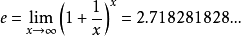设a>0，a≠1

方法一：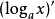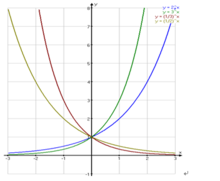指数函数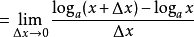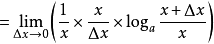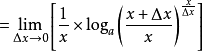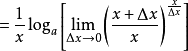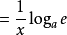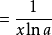特殊地，当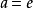时，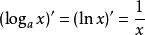。

方法二：

设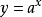，两边取对数ln y=xln a

两边对x求导：y'/y=ln a，y'=yln a=a^xln a

特殊地，当a=e时，y'=（a^x）'=（e^x）'=e^xln e=e^x。

eº=1

运算性质

编辑

性质

一般地，如果a（a>0，且a≠1）的b次幂等于N，那么数b叫做以a为底N的对数，记作logaN=b，其中a叫做对数的底数，N叫做真数。

底数则要>0且≠1 真数>0

并且，在比较两个函数值时：

如果底数一样，真数越大，函数值越大。（a>1时）

如果底数一样，真数越小，函数值越大。（0<a<1时）

当a>0且a≠1时，M>0，N>0，那么：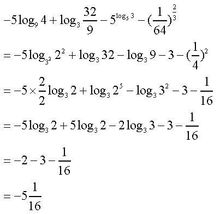对数函数化简问题

和差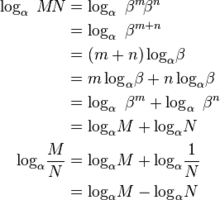和差

换底公式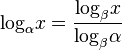换底公式

推导：设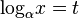换底公式

所以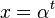换底公式

两边取对数，则有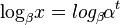换底公式

即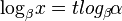换底公式

又因为换底公式

所以换底公式

指系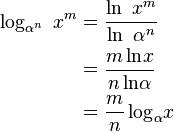指系

互换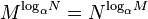互换

倒数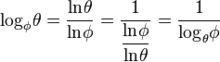倒数

链式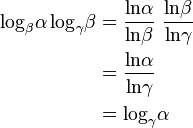链式 

表达方式

编辑

（1）常用对数：lg（b）=log10b（10为底数）。

（2）自然对数：ln（b）=logeb（e为底数）。

e为无限不循环小数，通常情况下只取e=2.71828。

与指数的关系

编辑

同底的对数函数与指数函数互为反函数。

当a>0且a≠1时，ax=Nx=㏒aN。

关于y=x对称。

对数函数的一般形式为 y=㏒ax，它实际上就是指数函数的反函数（图象关于直线y=x对称的两函数互为反函数），可表示为x=ay。因此指数函数里对于a的规定（a>0且a≠1），右图给出对于不同大小a所表示的函数图形：关于X轴对称、当a>1时，a越大，图像越靠近x轴、当0<a<1时，a越小，图像越靠近x轴。

可以看到，对数函数的图形只不过是指数函数的图形的关于直线y=x的对称图形，因为它们互为反

展开全文• ## python绘制对数函数

千次阅读 2020-02-18 11:16:47
如何利用python的matplotlib模块绘制对数函数
python绘制对数函数a>1完整代码效果图a>1完整代码效果图对数函数vs指数函数完整代码效果图参考文献
上一期，我们在探讨对数函数性质的时候，我们画了几个非常漂亮的图，这一期，就把画图的python放上来，设对数函数为
$y=log_a x$
其中a>0，且a≠1。对应的指数函数为$y=a^x$。现在来分情况画出对数函数的图像
a>1
完整代码
# -*- coding: utf-8 -*-
"""
Created on Mon Feb 17 17:32:49 2020
project name:draw_logarithm_figure
@author: 帅帅de三叔
"""
import math
import numpy as np
import matplotlib.pyplot as plt
import mpl_toolkits.axisartist as axisartist #导入坐标轴加工模块
plt.rcParams['font.sans-serif']=['SimHei']
plt.rcParams['axes.unicode_minus']=False

fig=plt.figure(figsize=(6,4)) #新建画布
ax=axisartist.Subplot(fig,111) #使用axisartist.Subplot方法创建一个绘图区对象ax

def logarithm_func(x, a=1/2): #定义指数函数
y=math.log(x, a)
return y

X=np.linspace(0.01, 4, 100) #构造自变量组
Y=[logarithm_func(x) for x in X] #求函数值
ax.plot(X, Y, label=r'$0<a<1$') #绘制指数函数
ax.scatter(1, 0, color='red')
plt.legend()
plt.show()

print(max(X), max(Y)) #测试一下自变量最大值和因变量最大值，为后面的坐标轴设置依据
ax.axis[:].set_visible(False) #隐藏原来的实线矩形
ax.axis["x"]=ax.new_floating_axis(0, 0, axis_direction="bottom") #添加x轴
ax.axis["y"]=ax.new_floating_axis(1, 0, axis_direction="bottom") #添加y轴

ax.axis["x"].set_axisline_style("-|>", size=1.0) #给x坐标轴加箭头
ax.axis["y"].set_axisline_style("-|>", size=1.0) #给y坐标轴加箭头

ax.annotate(s='x', xy=(max(X), 0), xytext=(max(X)+1, 0.3)) #标注x轴
ax.annotate(s='y', xy=(0, 1.0), xytext=(-0.2, max(Y)+1)) #标注y轴

plt.xlim(-1, 5) #设置横坐标范围
plt.ylim(-5, 3) #设置纵坐标范围
X_lim=np.arange(int(min(X)), max(X)+1, 1)
ax.set_xticks(X_lim) #设置x轴刻度
Y_lim=np.arange(-3, max(Y)+1, 1)
ax.set_yticks(Y_lim) #设置y轴刻度
ax.annotate(s=r'$y=log_a x$',xy=(1, 1), xytext=(1, 1)) #r'$y=(\frac{1}{2})^x$'

效果图a>1
完整代码
# -*- coding: utf-8 -*-
"""
Created on Mon Feb 17 17:32:49 2020
project name:draw_logarithm_figure
@author: 帅帅de三叔
"""
import math
import numpy as np
import matplotlib.pyplot as plt
import mpl_toolkits.axisartist as axisartist #导入坐标轴加工模块
plt.rcParams['font.sans-serif']=['SimHei']
plt.rcParams['axes.unicode_minus']=False

fig=plt.figure(figsize=(6,4)) #新建画布
ax=axisartist.Subplot(fig,111) #使用axisartist.Subplot方法创建一个绘图区对象ax

def logarithm_func(x, a=2): #定义指数函数
y=math.log(x, a)
return y

X=np.linspace(0.01, 4, 100) #构造自变量组
Y=[logarithm_func(x) for x in X] #求函数值
ax.plot(X, Y, label=r'$a>1$') #绘制指数函数
ax.scatter(1, 0, color='red')
plt.legend()
plt.show()

print(max(X), max(Y)) #测试一下自变量最大值和因变量最大值，为后面的坐标轴设置依据
ax.axis[:].set_visible(False) #隐藏原来的实线矩形
ax.axis["x"]=ax.new_floating_axis(0, 0, axis_direction="bottom") #添加x轴
ax.axis["y"]=ax.new_floating_axis(1, 0, axis_direction="bottom") #添加y轴

ax.axis["x"].set_axisline_style("-|>", size=1.0) #给x坐标轴加箭头
ax.axis["y"].set_axisline_style("-|>", size=1.0) #给y坐标轴加箭头

ax.annotate(s='x', xy=(max(X), 0), xytext=(max(X)+1, 0.3)) #标注x轴
ax.annotate(s='y', xy=(0, 1.0), xytext=(-0.2, max(Y)+1)) #标注y轴

plt.xlim(-1, 5) #设置横坐标范围
plt.ylim(-5, 3) #设置纵坐标范围
X_lim=np.arange(int(min(X)), max(X)+1, 1)
ax.set_xticks(X_lim) #设置x轴刻度
Y_lim=np.arange(-3, max(Y)+1, 1)
ax.set_yticks(Y_lim) #设置y轴刻度
ax.annotate(s=r'$y=log_a x$',xy=(1, 1), xytext=(1, 1)) #r'$y=(\frac{1}{2})^x$'

效果图两个代码对比，可以看到，仅改了参数a的初始值和label标签，因为两个函数的定义域和值域是一致的。
对数函数vs指数函数
这两个函数虽然互为反函数，但是定义域和值域不一致，所以需要在定义函数的时候给出不同的自变量取值范围，否则会出现math domain error 。
完整代码
# -*- coding: utf-8 -*-
"""
Created on Tue Feb 18 08:49:21 2020
project name:logarithm_vs_exponential
@author: 帅帅de三叔
"""
import math
import numpy as np
import matplotlib.pyplot as plt
import mpl_toolkits.axisartist as axisartist #导入坐标轴加工模块
plt.rcParams['font.sans-serif']=['SimHei']
plt.rcParams['axes.unicode_minus']=False

fig=plt.figure(figsize=(6,4)) #新建画布
ax=axisartist.Subplot(fig,111) #使用axisartist.Subplot方法创建一个绘图区对象ax

def logarithm_func(x, a=2): #定义指数函数
y=math.log(x, a)
return y

def exponential_func(x, a=2): #定义指数函数
y=math.pow(a, x)
return y

X=np.linspace(0.01, 4, 100) #构造自变量组
Y=[logarithm_func(x) for x in X] #求函数值
ax.plot(X, Y, label='对数函数') #绘制指数函数
ax.scatter(1, 0, color='red')

X1=np.linspace(-4, 4, 100)
Y1=[exponential_func(x) for x in X1]
ax.plot(X1, Y1, label='指数函数')
ax.scatter(0, 1, color='red')

ax.plot(X1, X1, color='green', label=r'$y=x$')
plt.legend(loc=2)
plt.show()

print(max(X), max(Y)) #测试一下自变量最大值和因变量最大值，为后面的坐标轴设置依据
ax.axis[:].set_visible(False) #隐藏原来的实线矩形
ax.axis["x"]=ax.new_floating_axis(0, 0, axis_direction="bottom") #添加x轴
ax.axis["y"]=ax.new_floating_axis(1, 0, axis_direction="bottom") #添加y轴

ax.axis["x"].set_axisline_style("-|>", size=1.0) #给x坐标轴加箭头
ax.axis["y"].set_axisline_style("-|>", size=1.0) #给y坐标轴加箭头

ax.annotate(s='x', xy=(max(X), 0), xytext=(max(X)+1, 0.3)) #标注x轴
ax.annotate(s='y', xy=(0, 1.0), xytext=(-0.2, 5)) #标注y轴

plt.xlim(-5, 5) #设置横坐标范围
plt.ylim(-5, 5) #设置纵坐标范围
X_lim=np.arange(-4, 4, 1)
ax.set_xticks(X_lim) #设置x轴刻度
Y_lim=np.arange(-4, 4, 1)
ax.set_yticks(Y_lim) #设置y轴刻度

效果图只需要在定义指数函数和对数函数的时候给a赋予不同的参数便可出现不同的形状，下图是a=1/2 时的效果图如果你不会写代码或者还有什么不懂的欢迎来“三行科创”微信公众号留言，同时交流群免费向大家开放，入群讲缘分。
参考文献
1，https://blog.csdn.net/zengbowengood/article/details/104338878
2，https://blog.csdn.net/zengbowengood/article/details/102862072
3，https://blog.csdn.net/zengbowengood/article/details/104360150
4，https://blog.csdn.net/zengbowengood/article/details/104260155
5，https://matplotlib.org/api/_as_gen/matplotlib.pyplot.html
6，https://blog.csdn.net/qq_17528659/article/details/82152530展开全文python matplotlib
• 指数函数，幂函数，对数函数为高等数学中的初等函数 指数函数 指数函数公式为，其函数增长性如下： 指数函数的单调性是递增的，当x=0时，不管a为任何，其为1。当a大于1时，随着a越大，其函数值增长越快 在...
指数函数，幂函数，对数函数为高等数学中的初等函数

指数函数

指数函数公式为，其函数增长性如下：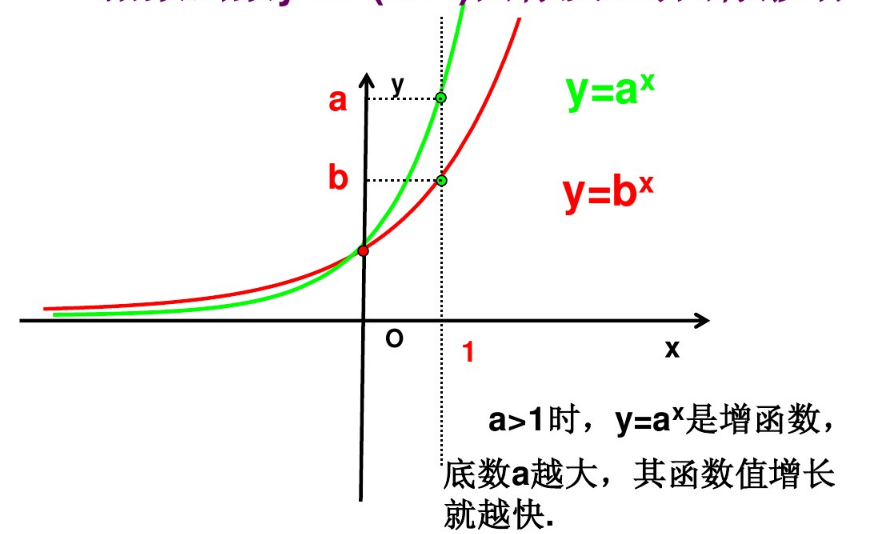指数函数的单调性是递增的，当x=0时，不管a为任何值，其值为1。当a大于1时，随着a越大，其函数值增长越快

在x>0部分，a>b其y值也是随着>

在x<0部分 当a>b是，其<<1

对数函数

对数函数表达式为：,其函数图像为如下：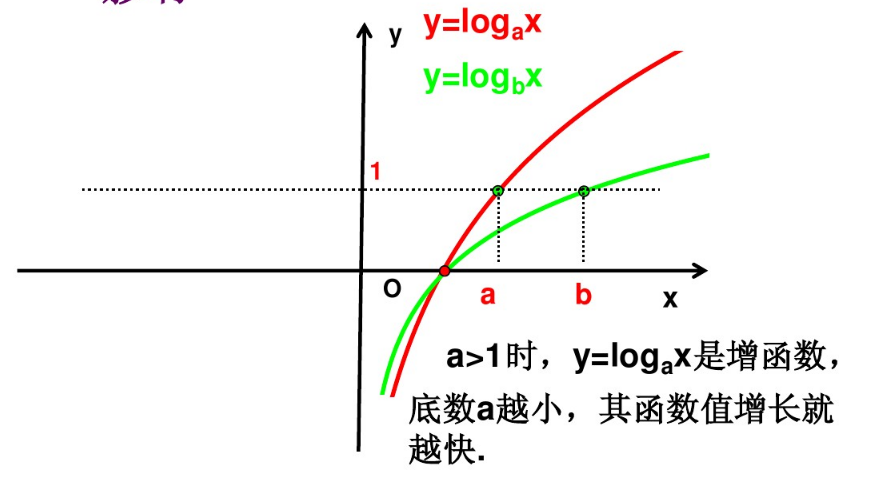当x等于1时 y为0，

当x<1时，其y值小于0

当x >1时，其值大于0

对数函数为单调递增的，当a>1时，随着地鼠a越小，其函数增长值越快

当x> 1时， a<b，>

当x<1时， a<b ,<

幂函数

幂函数表达式为,其图像如图：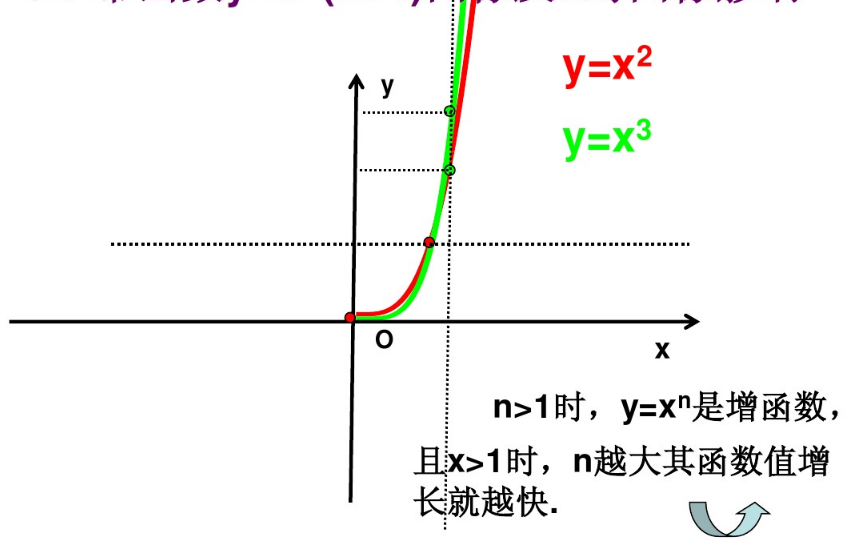对数函数为单调递增的，当n大于1时且x大于1时， n越大其函数值越大

比较三个函数,,增长快慢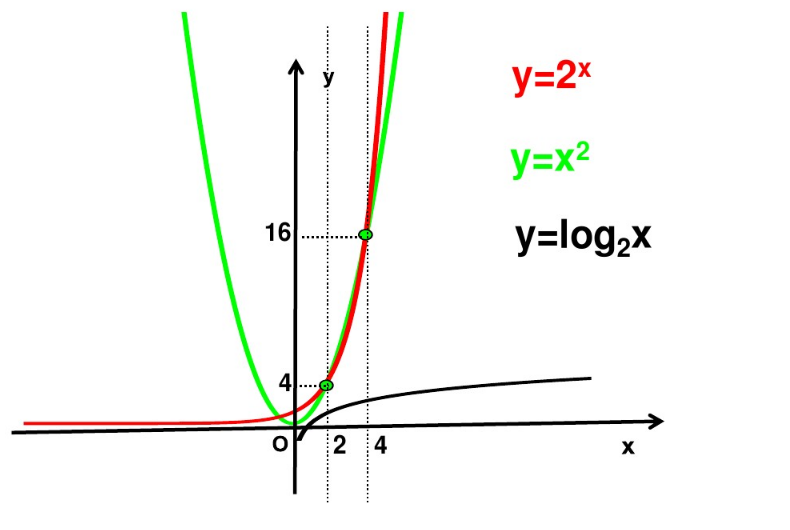增长最慢，幂函数和指数函数快慢交替进行

在x(0.2)区间,幂函数比指函数增长较快

在（4，+）指数函数比幂函数增长较快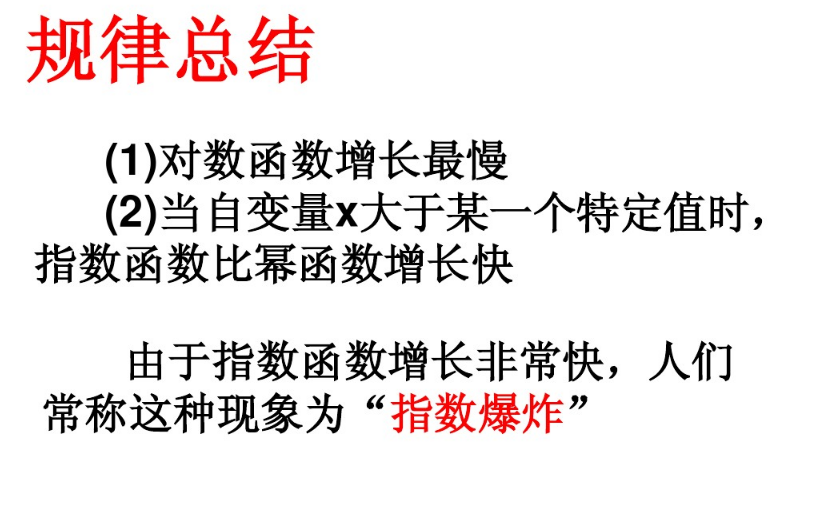展开全文高等数学
•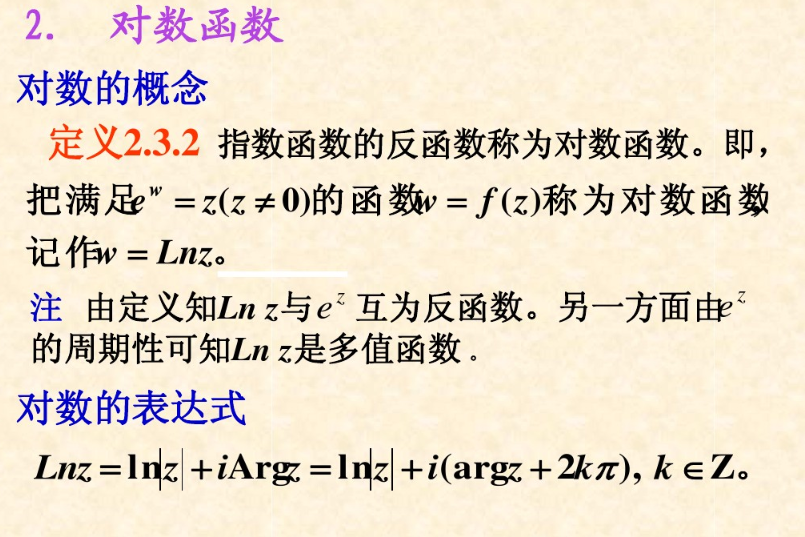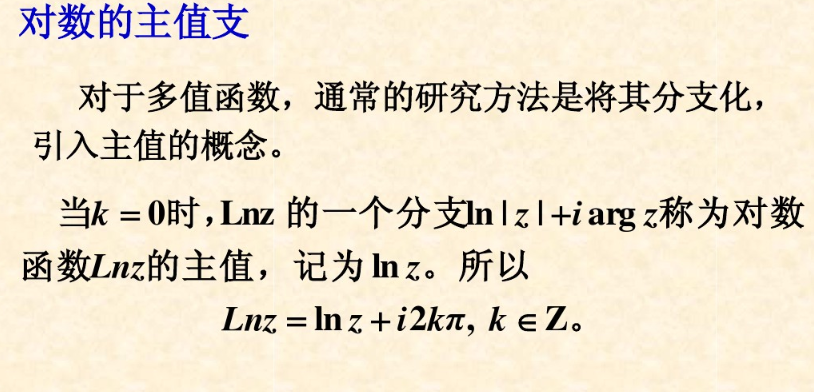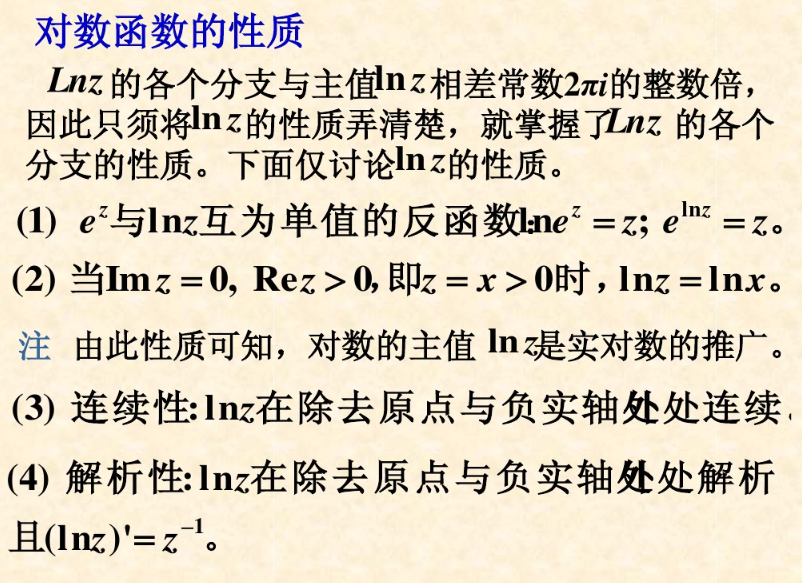展开全文• ## 对数函数及运算

千次阅读 2018-01-29 18:36:58
一般地，对数函数以幂（真数）为自变量，指数为因变量，底数为常量的函数。 对数函数是6类基本初等函数之一。其中对数的定义： 如果ax=N（a>0，且a≠1），那么数x叫做以a为底N的对数，记作x=logaN，读作以a为...
• 之后 的导数可以根据对数的导数推导如下： 令 , 所以 ，俩边求导, 根据复合函数求导法则为： 或者记住 的导数，用复合函数求导推 的导数。但是个人觉得这种做法太讨巧了，而且我也不是总能记住其中一个...指数函数 定义
• python对数函数Logarithms are used to depict and represent large numbers. The log is an inverse of the exponent. This article will dive into the Python log() functions. The logarithmic functions of ...
• log(真数（幂）):以e为底的对数函数 log10():以10为底的对数函数 如果要求自定义以a为底，log n 的，则需要使用换底公式即 log an = log(n) / log(a) 例题：1、计算公式的(对数)（10分） 题目内容： 输入x、a...抽象代数
• 指数函数的性质 　先来复习一下中学的课程： 指数函数的导数 　对f(x) = ax求导： 　ax右侧的那个极限似乎没有办法继续简化了，如果这个极限看作关于a的函数（之所以将极限看作关于a的函数，是因为在这个...数学 指数函数
• ## c++对数函数 log() 操作

万次阅读 多人点赞 2018-03-23 22:49:36
首先要知道exp（）函数exp（n）为e^n次方；另外log函数包括两种函数 一种以e为低的log（）函数另一种为以10为底的log 10（）函数；具体用法见下面这个小程序#include&lt;iostream&gt; #include&lt;...
• 有错的地方，或者不同意见的，烦请留言，或者发邮箱。 ... ————————————————————————————————— python是用来导入模块的...所以使用函数，需要使用math模块。 [gyz@archlinux job]...python
• 可以使用对数函数的通用计算函数：Math.Log（），但是关于这个函数的使用网上很多都搞错了，第一个参数是想，第二个参数是底。 为此，我做了如下试验， 再次验证了第二个参数是底！另外，如何一个参数的话...
• 1、C语言中的对数函数  头文件：#include  格式：  ①double log (double x);  log() 函数返回以 e 为底的对数值，即数学中的lnx；  如果 x 为负数或 0，则会发生错误并设置 errno 。  错误代码：EDOM：参数...
• exp（n）为e^n次方； 另外log函数包括两种函数 一种以e为低的log（）函数 另一种为以10为底的log 10（）函数； 具体用法见下面这个小程序 #include&lt;iostream&gt; #include...
• Iterated Logarithm Function 多重对数函数 —— 通俗地讲，就是使得lg * n ≦ 1 的最小 i 。 We use the notation lg* n (read "log star of n") to denote the iteratedlogarithm, which is defined ...迭代
• 大于零的数进行开方运算，当开方次数无限增加时，开方无限接近1)，当然开方不是乱用的，必需有理论或已有公式的支持，这个公式就是：，其实这个算法本身并不会使对数运算获得本质的提升，提速的根本是开平方有快速...大数运算 GMP大数库
• #define e 2.71828 //使用define函数给e定义初值 main() { int x; printf("请你输入x的\n"); scanf("%d",&amp;x); if(0&lt;=x&amp;&amp;x&lt;20) printf("y=%d
• ## 对数似然函数理解

千次阅读 2019-09-19 21:08:20
对数似然函数（log likelihood） 机器学习里面，对模型的训练都是对Loss function进行优化，在分类问题中，我们一般使用最大似然估计（Maximum likelihood estimation）来构造损失函数。对于输入的x，其对应的类...
• 深度学习以及机器学习中都会用到SoftMax函数，交叉熵损失函数与熵，对数似然函数等一些数学方面的知识，此文作为个人学习笔记。 1.softmax函数 （1）定义 多分类问题中，我们可以使用SoftMax函数，对输出的归一...
• 最大熵模型中的对数似然函数的解释 最近在学习最大熵模型，看到极大似然估计这部分，没有看明白条件概率分布p(y|x)p(y|x)p(y|x)的对数似然函数。上网查了很多资料都没有一个合理的解释。基本直接给出对数似然函数的...极大似然估计
• 当时，，相当于对log(f)进行扩展延伸，此时，如果扩展值函数log(f)是凹函数，则f是对数凹的。 函数，其定义域时凸集，且，函数对数凹的当且仅当 特别的，当时，上式 即对数凹函数在两点之间中间的函数值不...
• 1，最大似然损失函数（Likelihood loss） 常用在分类问题上。形式上是把每一个预测的概率相乘，得到一个损失。 例如：对一组样本的预测为True(1)概率为[0.4, 0.6, 0.9, 0.1]，它们的真实分别为[0, 1, 1, 0]，...
• 在数字逻辑设计中，本节介绍绝对值运算函数如何用Verilog硬件描述语言来实现，本质上是补码的问题。 而取对数问题，可以归结为移位寄存器的问题。...二、取对数函数log以2为低的整数） 三、取整函数
• 似然函数 似然函数是一种关于统计模型参数的函数，给定输出y时，关于参数x的似然函数...负对数似然函数 高斯分布的概率密度函数 对应负对数似然函数 泊松分布的概率密度函数 对应负对数似然函数 ...
• C语言中、Qt中，都没有以任意为底数的对数函数，所以log5 ^3 [以5为底]是没有的。但是可以用logx(y)=ln(y)/ln(x)来代替，修改代码如下 1、Qt中对数，通过自然对数qLn()转换一下,logx(y)=ln(y)/ln(x) ...c++ Qt 数学 c语言
• ## numpy对数组求平均时如何忽略nan值

万次阅读 多人点赞 2018-12-13 09:19:59
前言：在对numpy数组平均np.mean()或者数组中最大最小值np.max()/np.min()时，如果数组中有nan,此时求得的结果为：nan，那么该如何忽略其中的nan呢？此时应该用另一个方法：np.nanmean(),np.nanmax(),np.nanmin...python nan
• 二次代价函数的局限性 首先来说一下二次代价函数的局限性，看下面这张图： 假设现在使用的激活函数是sigmoid，并且代价函数是二次代价函数，我们收敛得目标是1，那么A点的梯度比较大，B点由于离目标比较近梯度小，......